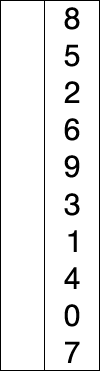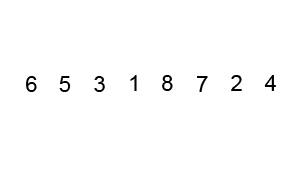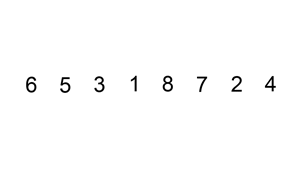# Selection Sort, Bubble Sort, and Insertion Sort

Here's an overview of introductory sorting algorithms such as selection sort, bubble sort, and insertion sort.

We'll cover the following

## What is sorting?

Sorting is any process of arranging items systematically. In computer science, sorting algorithms put elements of a list in a certain order.

The most frequently used orders are numerical (according to numbers) and lexicographical (according to letters). Efficient sorting is important for optimizing the efficiency of other algorithms, which require sorted input or the sort of a given input as part of their process.

### Formal definition

A list or array is sorted in ascending order if $\forall$ $i$, such that $i \leq n-1$, $A[i]\leq A[i+1]$. Similarly, a list or array is sorted in descending order if $\forall$ $i$, such that $i \leq n-1$, $A[i]\geq A[i+1]$.

## Sorting algorithms

Let’s discuss a few sorting algorithms:

### Selection sort algorithm

This algorithm works by repeatedly finding the minimum element in the list and placing it at the beginning. This way, the algorithm maintains two lists:

1. the sublist of already-sorted elements, which is filled from left to right
2. the sublist of the remaining unsorted elements that need to be sorted

In other words, this algorithm works by iterating over the list and swapping each element with the minimum (or maximum) element found in the unsorted list with that in the sorted list.

Look at the illustration for a clearer picture.

Also, here is the code:def selection_sort(lst):    """    Selection sort function    :param lst: List of integers    """    # Traverse through all lst elements    for i in range(len(lst)):        # Find the minimum element in unsorted lst        min_index = i        for j in range(i + 1, len(lst)):            if lst[min_index] > lst[j]:                min_index = j        # Swap the found minimum element with the first element        lst[i], lst[min_index] = lst[min_index], lst[i]# Driver code to test aboveif __name__ == '__main__':    lst = [3, 2, 1, 5, 4]    selection_sort(lst)  # Calling selection sort function    # Printing Sorted lst    print("Sorted lst: ", lst)

#### Time complexity

The time complexity of this code is in $O(n^2)$ because finding a minimum number in the list requires iterating over the entire list for each element of the given list. The quadratic time complexity makes it impractical for large inputs.

### Bubble sort algorithm

This is another famous sorting algorithm also known as ‘sinking sort’. It works by comparing adjacent pairs of elements and swapping them if they are in the wrong order. This is repeated until the list is sorted.

Think of it this way: a bubble passes over the list, ‘catches’ the maximum/minimum element, and brings it over to the right side.def bubble_sort(lst):    """    Bubble sort function    :param lst: lst of unsorted integers    """    # Traverse through all list elements    for i in range(len(lst)):        # Last i elements are already in place        for j in range(0, len(lst) - i - 1):            # Traverse the list from 0 to size of lst - i - 1            # Swap if the element found is greater than the next element            if lst[j] > lst[j + 1]:                lst[j], lst[j + 1] = lst[j + 1], lst[j]# Driver code to test aboveif __name__ == '__main__':    lst = [3, 2, 1, 5, 4]    bubble_sort(lst)  # Calling bubble sort function    print("Sorted list is: ", lst)

#### Time complexity

The time complexity of this code is $O(n^2)$. Again, this algorithm is not very efficient.

### Insertion sort

Insertion sort is another famous sorting algorithm and works the way you would naturally sort in real life.

It iterates over the given list, figures out what the correct position of every element is, and inserts it there.def insertion_sort(lst):    """    Insertion sort function    :param lst: lst of unsorted integers    """    # Traverse through 1 to len(lst)    for i in range(1, len(lst)):        key = lst[i]        # Move elements of lst greater than key, to one position ahead        j = i - 1        while j >= 0 and key < lst[j]:            lst[j + 1] = lst[j]            j -= 1        lst[j + 1] = key# Driver code to test aboveif __name__ == '__main__':    lst = [3, 2, 1, 5, 4]    insertion_sort(lst)  # Calling insertion sort function    print("Sorted list is: ", lst)
The algorithm is $O(n^2)$, which, again, makes it a poor choice for large input sizes. However, notice that the complexity is actually $n^2$ only when the input list is sorted in reverse. So, the ‘best-case’ complexity (when the list is sorted in the correct order) is $\Omega(n)$.
Also, note that all of these algorithms are in-place, hence their space complexity is in $O(1)$.